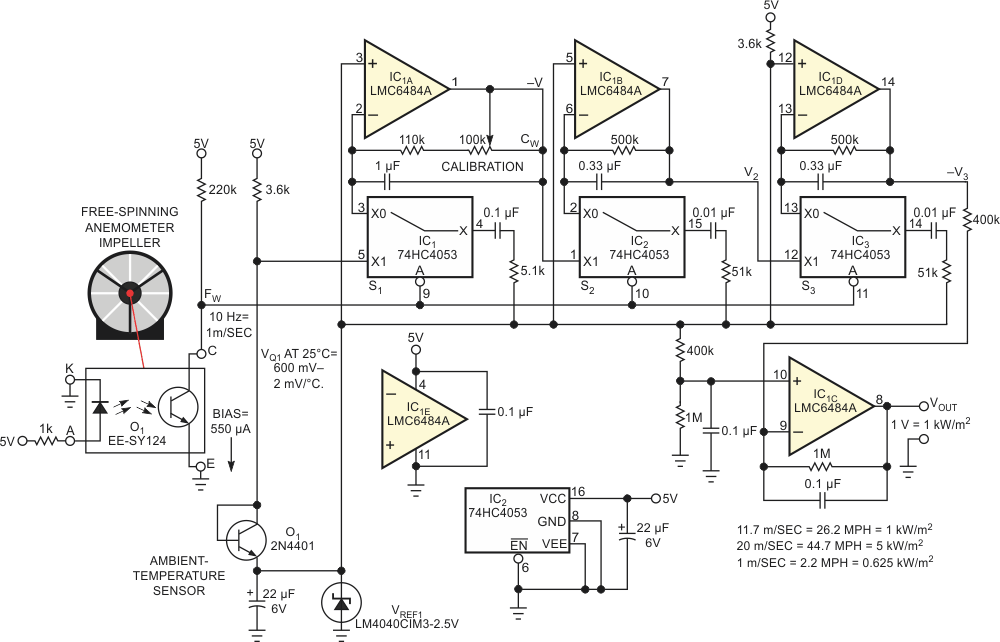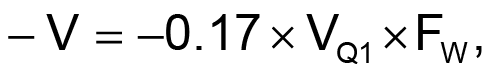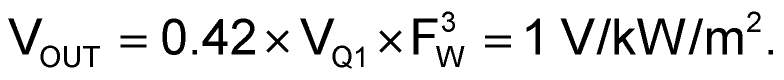# Linear wind-power meter compensates for temperature

## Texas Instruments CD74HC4053 LM4040 LMC6484

Stephen Woodward

EDN

The rise of interest in renewable energy created by soaring fossil-fuel costs and global-warming fears has created a matching interest in associated support and demonstration instrumentation. This Design Idea hops on that bandwagon with the ability to directly and conveniently measure an important renewable-energy source: wind power. Handy for quick and easy preliminary evaluation of potential wind-turbine sites, it includes a wind-speed transducer, comprising an optically sensed vane anemometer, and a temperature sensor, comprising a diode-connected transistor (Figure 1). These components interface with a hybrid digital/analog-computation circuit. In combination, they provide a real-time, linear, temperature-compensated readout of wind-power density.Figure 1. This meter circuit uses a free-spinning anemometer and a diode-connected transistor temperature sensor to measure the available wind power for “green” power generation.

The power-generation potential of wind is

½×air density (kg/m3)×air speed (m/sec)3.

To compute it, therefore, requires estimating air density, which is inversely proportional to absolute temperature; measuring air speed; and calculating a cube.

Here’s how the wind-power meter does it. Diode-connected Q1 has a bias of 550 µA for a 25 °C (298K), base-to-emitter voltage of approximately 600 mV and a temperature coefficient of –2 mV/°C. Thus, Q1 is a voltage reference that tracks the approximate ideal-gas-law temperature dependence of air density: –0.3%/°C. Meanwhile, optical sensor O1 works with a free-spinning anemometer impeller to produce wind-speed-proportional frequency:

FW = 10 Hz/m/sec.

Conversion of VQ1 and FW into a 1-mV = 1 W/m2 output signal is then the function of the third-order X×Y×Z-multiplying behavior of three cascaded CMOS-switch FVC (frequency-to-voltage-converter) charge pumps: S1, S2, and S3.

FVC S1/IC1A generates a negative voltage ofFVC S2/IC1B generates

V2 = –V × FW = 0.17 ×VQ1 × FW2;

and FVC S3/IC1D generates

–V3 = –0.17 × VQ1 × FW3.

Finally, differential inverter IC1C shifts and scales –V3 to outputYou can conveniently calibrate the wind-power meter in an automobile being driven on a windless day at a constant speed of 18.6 m/sec = 41.5 mph = 66.8 kph. With the anemometer exposed to the external slip-stream, adjust the calibration trimming potentiometer for an output voltage of 4 V or, for better accuracy, to the voltage that the following formula that accommodates true air density yields:

VOUT = 1.14 V × air-pressure millibar/(273 + ambient temperature °C).

## Materials on the topic

EDN